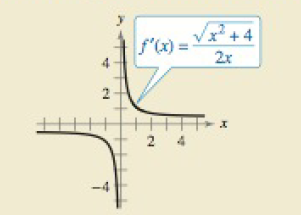Chapter 8.4, Problem 46E

Chapter
Section
Textbook Problem

How do you see it?Use the graph of f 1 shown in the figure to answer the following(a). Identify the open interval(s) on which the graph of f is increasing or decreasing. explain.(b). Identify the open intervals(s) on which the graph of f is concave upward or downward. explain.

(a)

To determine
Whether the open interval(s) on which the graph of ‘f’ is increasing or decreasing.

Explanation

Given:

Refer to the question for graph.

Solution:

f is increasing on the open interval 0<x<=(0,)._

f is decreasing on the open interval <x<0=(,0)

(b)

To determine
Whether the open interval(s) on which the graph of ‘f ‘is concave upward or downward.

Still sussing out bartleby?

Check out a sample textbook solution.

See a sample solution

The Solution to Your Study Problems

Bartleby provides explanations to thousands of textbook problems written by our experts, many with advanced degrees!

Get Started

Find the limit: limx3x2+x12x3

Calculus: An Applied Approach (MindTap Course List)

Factor: 60ax12a

Elementary Technical Mathematics

log27 9 =

Study Guide for Stewart's Single Variable Calculus: Early Transcendentals, 8th

Multiply: 12.3457.86 .

Mathematics For Machine Technology